# Locating and Decoding EAN-13 Barcodes using Python and OpenCV

The International Article Number (also known as European Article Number or EAN) is a standard describing a barcode symbology and numbering system used in global trade to identify a specific retail product type. The most commonly used EAN standard is the thirteen-digit EAN-13. 1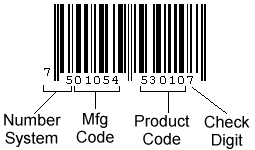The 13-digit EAN-13 number consists of four components:

• GS1 prefix
• Manufacturer code
• Product code
• Check digit

An EAN-13 barcode has 95 areas (also known as modules) of equal width. Each area can be either white (represented here as 0) or black (represented as 1). Continuous areas form a black or white bar. There are 59 bars in an EAN-13 barcode.

From left to right, there are:

• 3 areas for the start guard (101)
• 42 left-hand areas (seven per digit) to encode the 2nd to 7th digits. Each digit is represented by four bars. The first digit can be then inferred from the 6 digits.
• 5 areas for the center guard (01010)
• 42 right-hand areas (seven per digit) to encode the 8th to 13th digits. Each digit is represented by four bars.
• 3 areas for the end guard (101)

The encoding of the digit can be known by looking up the following table.

Digit Left-hand (Odd) Left-hand (Even) Right-hand
0 0001101 0100111 1110010
1 0011001 0110011 1100110
2 0010011 0011011 1101100
3 0111101 0100001 1000010
4 0100011 0011101 1011100
5 0110001 0111001 1001110
6 0101111 0000101 1010000
7 0111011 0010001 1000100
8 0110111 0001001 1001000
9 0001011 0010111 1110100

The left-hand digits have a parity property which is odd and even. The initial digit can be inferred by checking the following table.

First digit The parity of the 6 left-hand digits
0 OOOOOO
1 OOEOEE
2 OOEEOE
3 OOEEEO
4 OEOOEE
5 OEEOOE
6 OEEEOO
7 OEOEOE
8 OEOEEO
9 OEEOEO

In the following part, we will try to decode and detect EAN-13 barcodes using Python and OpenCV.

## Decoding EAN-13 Barcodes

Based on the specification of EAN-13, we can create an EAN-13 barcode decoder.

Here is a test barcode images generated with an online tool: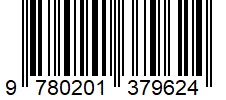### Get the Data Sequence

Since it is a generated image, we can directly read the barcode data from it.

1. Create a thresholded image.

`````` img = cv2.imread("generated.jpg")
gray = cv2.cvtColor(img, cv2.COLOR_BGR2GRAY)
ret, thresh =cv2.threshold(gray, 200, 255, cv2.THRESH_BINARY + cv2.THRESH_OTSU)
``````
2. The value of the white pixels of the thresholded images is 255 and the black pixels 0. We need to invert it and replace 255 with 1 to conform to the 0 and 1 pattern. Only one line of the barcode is needed and here, we use the middle line.

`````` thresh = cv2.bitwise_not(thresh)
line = thresh[int(img.shape/2)]
for i in range(len(line)):
if line[i] == 255:
line[i] = 1
``````
3. Read the 95 areas and detect the module size. The module size is the length of the smallest bar.

`````` def read_bars(line):
bars = []
current_length = 1
for i in range(len(line)-1):
if line[i] == line[i+1]:
current_length = current_length + 1
else:
bars.append(current_length * str(line[i]))
current_length = 1
#remove quite zone
bars.pop(0)
return bars

def detect_module_size(bars):
size = len(bars)
for bar in bars:
size = min(len(bar),size)
return size

``````
4. Get the data string.

`````` def array_as_string(array, module_size):
s = ""
for value in array:
s = s + str(value)
s=s.replace("1"*module_size,"1")
s=s.replace("0"*module_size,"0")
print("Data string: " + s)
return s

data_string = array_as_string(line,module_size)
``````

The data string of the test image:

`````` 00000000000101011101100010010100111001001101001110011001010101000010100010011101001010000110110010111001010000000
``````

### Decode the Data

Now we can separate the data string by the fixed width of digits and guard markers and decode them according to the encoding table.

1. Decode the left half.

`````` def decode_left_bar_pattern(pattern):
left_pattern_dict = {}
left_pattern_dict["0001101"] = {"code":0,"parity":"O"}
left_pattern_dict["0100111"] = {"code":0,"parity":"E"}
left_pattern_dict["0011001"] = {"code":1,"parity":"O"}
left_pattern_dict["0110011"] = {"code":1,"parity":"E"}
left_pattern_dict["0010011"] = {"code":2,"parity":"O"}
left_pattern_dict["0011011"] = {"code":2,"parity":"E"}
left_pattern_dict["0111101"] = {"code":3,"parity":"O"}
left_pattern_dict["0100001"] = {"code":3,"parity":"E"}
left_pattern_dict["0100011"] = {"code":4,"parity":"O"}
left_pattern_dict["0011101"] = {"code":4,"parity":"E"}
left_pattern_dict["0110001"] = {"code":5,"parity":"O"}
left_pattern_dict["0111001"] = {"code":5,"parity":"E"}
left_pattern_dict["0101111"] = {"code":6,"parity":"O"}
left_pattern_dict["0000101"] = {"code":6,"parity":"E"}
left_pattern_dict["0111011"] = {"code":7,"parity":"O"}
left_pattern_dict["0010001"] = {"code":7,"parity":"E"}
left_pattern_dict["0110111"] = {"code":8,"parity":"O"}
left_pattern_dict["0001001"] = {"code":8,"parity":"E"}
left_pattern_dict["0001011"] = {"code":9,"parity":"O"}
left_pattern_dict["0010111"] = {"code":9,"parity":"E"}
return left_pattern_dict[pattern]

guard_pattern = "101"
center_guard_pattern = "01010"

begin_index = data_string.find(guard_pattern)+len(guard_pattern)
data_string_left = data_string[begin_index:-1]

left_codes = []
for i in range(6):
start_index = i*7
bar_pattern = data_string_left[start_index:start_index+7]
decoded = decode_left_bar_pattern(bar_pattern)
left_codes.append(decoded)
``````
2. Get the initial digit.

`````` def get_first_digit(left_codes):
parity_dict = {}
parity_dict["OOOOOO"] = 0
parity_dict["OOEOEE"] = 1
parity_dict["OOEEOE"] = 2
parity_dict["OOEEEO"] = 3
parity_dict["OEOOEE"] = 4
parity_dict["OEEOOE"] = 5
parity_dict["OEEEOO"] = 6
parity_dict["OEOEOE"] = 7
parity_dict["OEOEEO"] = 8
parity_dict["OEEOEO"] = 9
parity = ""
for code in left_codes:
parity = parity + code["parity"]
return parity_dict[parity]
``````
3. Decode the right half.

`````` def decode_right_bar_pattern(pattern):
right_pattern_dict = {}
right_pattern_dict["1110010"] = {"code":0}
right_pattern_dict["1100110"] = {"code":1}
right_pattern_dict["1101100"] = {"code":2}
right_pattern_dict["1000010"] = {"code":3}
right_pattern_dict["1011100"] = {"code":4}
right_pattern_dict["1001110"] = {"code":5}
right_pattern_dict["1010000"] = {"code":6}
right_pattern_dict["1000100"] = {"code":7}
right_pattern_dict["1001000"] = {"code":8}
right_pattern_dict["1110100"] = {"code":9}
return right_pattern_dict[pattern]

center_index = data_string_left.find(center_guard_pattern)+len(center_guard_pattern)
data_string_left = data_string_left[center_index:-1]

right_codes = []
for i in range(6):
start_index = i*7
bar_pattern = data_string_left[start_index:start_index+7]
decoded = decode_right_bar_pattern(bar_pattern)
right_codes.append(decoded)
``````
4. Check if the code is valid.

We can calculate the checksum and see if it matches the final digit.

`````` def verify(ean13):
weight = [1,3,1,3,1,3,1,3,1,3,1,3,1,3]
weighted_sum = 0
for i in range(12):
weighted_sum = weighted_sum + weight[i] * int(ean13[i])
weighted_sum = str(weighted_sum)
checksum = 0
units_digit = int(weighted_sum[-1])
if units_digit != 0:
checksum = 10 - units_digit
else:
checksum = 0
print("The checksum of "+ean13 + " is " + str(checksum))
if checksum == int(ean13[-1]):
print("The code is valid.")
return True
else:
print("The code is invalid.")
return False
``````

### Make the Decoding More Robust

The above decoding method has high requirements of image qualities. It cannot decode barcodes in the real world.

Here is a scan of a printed EAN-13 barcode and its thresholded images. We can see that the bars have many noises and the width of areas is affected by the printing.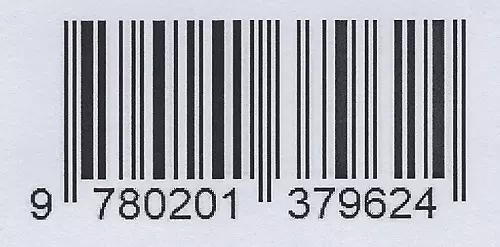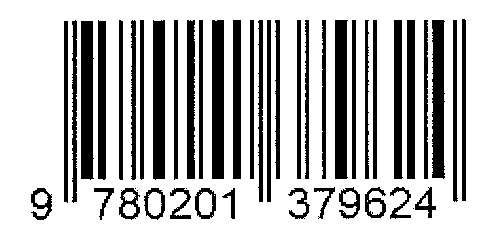For example, the left guard pattern should be 101 while in the scan, it is 1011, which makes it impossible to correctly detect where the barcode starts.

There are ways to improve the decoding like median blur, smooth and scanning every line. One of the ways which prove effective is using a similar edge distance algorithm to normalize the length of digit areas since they have a fixed width of 7.

## Detecting EAN-13 Barcodes

Let’s take a step further to detect barcodes in an image.

A basic detection method based on morphology and contours finding is used in this article.

Here is a sample image from the Artelab Medium Barcode 1D Collection: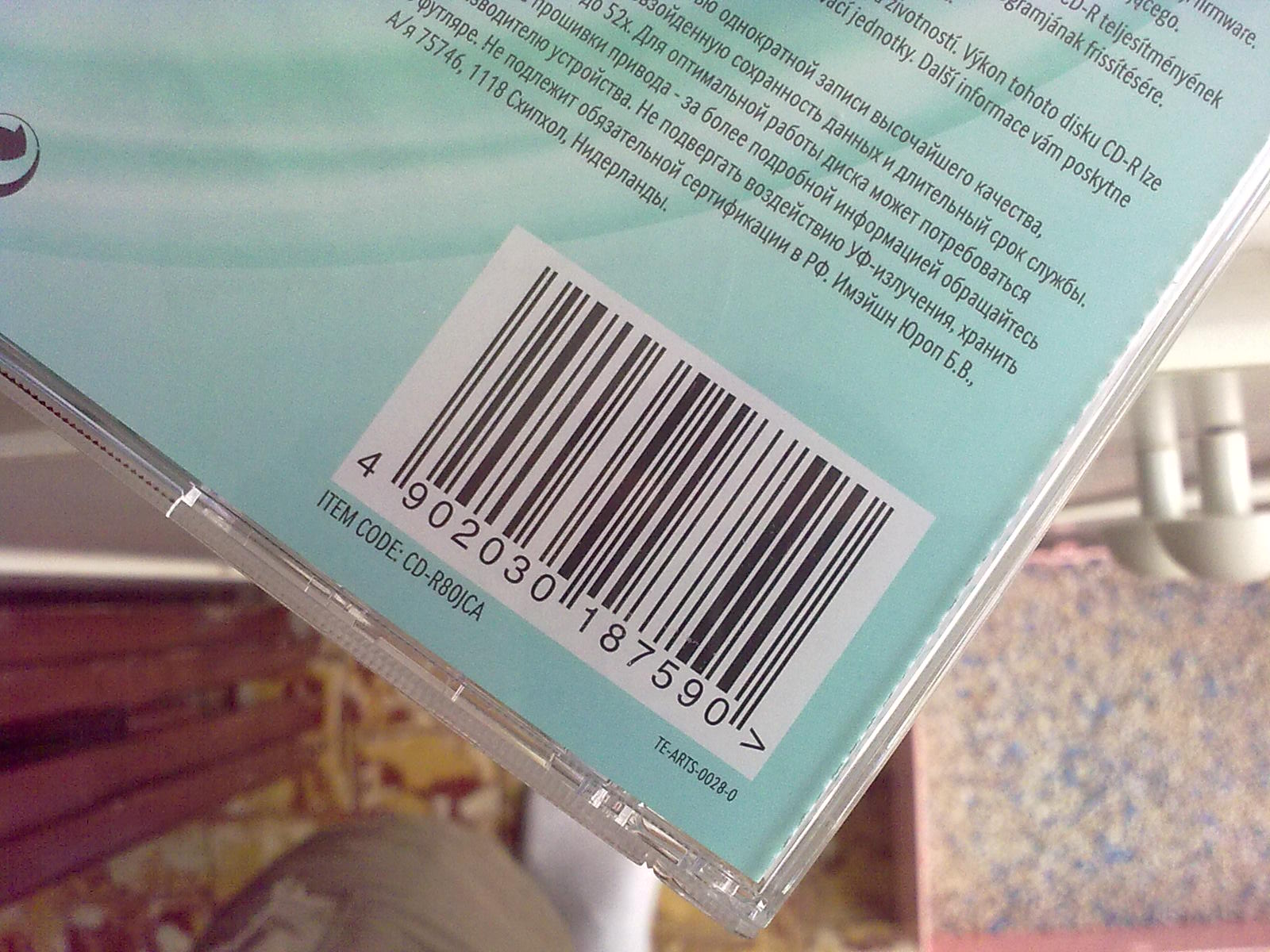We can see that the barcode has parallel lines, white background and quiet zones, making it very different from the rest of the content.

Let’s try to detect the barcode.

1. Resize the image for normalization.

`````` img = cv2.imread("05102009081.jpg")
scale_percent = 640/img.shape
width = int(img.shape * scale_percent)
height = int(img.shape * scale_percent)
dim = (width, height)
resized = cv2.resize(img, dim, interpolation = cv2.INTER_AREA)
``````
2. Create a thresholded image.

`````` gray = cv2.cvtColor(resized, cv2.COLOR_BGR2GRAY)
ret, thresh =cv2.threshold(gray, 200, 255, cv2.THRESH_BINARY + cv2.THRESH_OTSU)
``````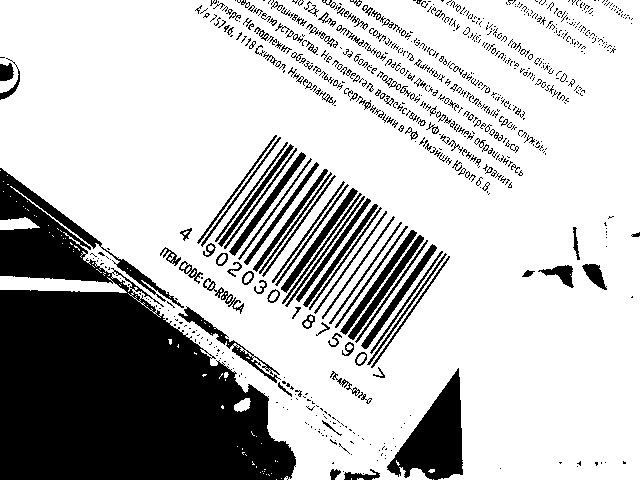3. Invert and dilate.

`````` thresh = cv2.bitwise_not(thresh)
kernel = np.ones((3, 20), np.uint8)
thresh = cv2.dilate(thresh, kernel)
``````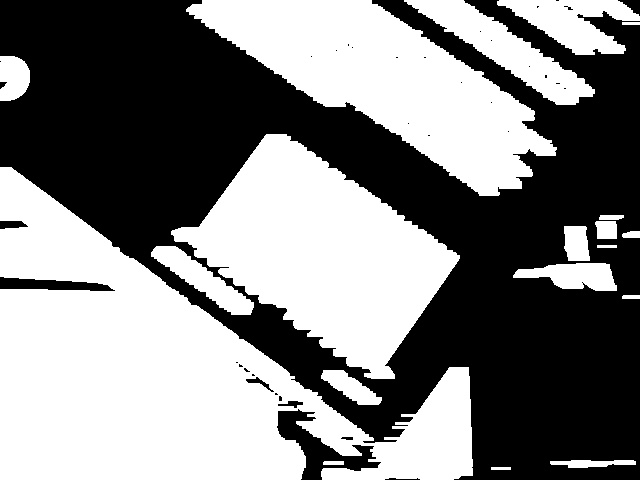4. Find contours and get the cropped and rotated candidate areas.

`````` def crop_rect(rect, box, img):
W = rect
H = rect
Xs = [i for i in box]
Ys = [i for i in box]
x1 = min(Xs)
x2 = max(Xs)
y1 = min(Ys)
y2 = max(Ys)

# Center of rectangle in source image
center = ((x1+x2)/2,(y1+y2)/2)
# Size of the upright rectangle bounding the rotated rectangle
size = (x2-x1, y2-y1)
# Cropped upright rectangle
cropped = cv2.getRectSubPix(img, size, center)

angle = rect
if angle!=90: #need rotation
if angle>45:
angle = 0 - (90 - angle)
else:
angle = angle
M = cv2.getRotationMatrix2D((size/2, size/2), angle, 1.0)

cropped = cv2.warpAffine(cropped, M, size)
croppedW = H if H > W else W
croppedH = H if H < W else W
# Final cropped & rotated rectangle
croppedRotated = cv2.getRectSubPix(cropped, (int(croppedW),int(croppedH)), (size/2, size/2))
return croppedRotated
return cropped

original_sized = cv2.resize(thresh, (img.shape,img.shape), interpolation = cv2.INTER_AREA)
contours, hierarchy = cv2.findContours(original_sized,cv2.RETR_TREE,cv2.CHAIN_APPROX_SIMPLE)
candidates = []
index = 0
for cnt in contours:
rect = cv2.minAreaRect(cnt)
box = cv2.boxPoints(rect)
box = np.int0(box)
cropped = crop_rect(rect,box,img)
width = cropped.shape
child_index = hierarchy[index]
#the min width of EAN13 is 95 pixel
if width>95:
has_overlapped = False
has_overlapped = True
if has_overlapped == False:
candidate = {"cropped": cropped, "rect": rect}
candidates.append(candidate)
index = index + 1
``````

We can get the following candidates. We can later send them to decode.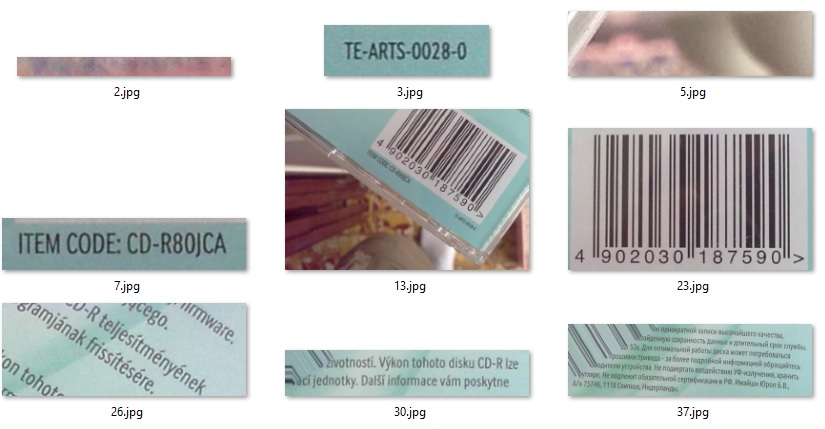## Put Things Together

Now, we can create an EAN-13 reader combining the detecting and decoding parts.

``````import decode as decoder
import detect as detector
import cv2
import numpy as np

def decode_image(image):
result_dict = {}
results = []

candidates = detector.detect(image)
for i in range(len(candidates)):
candidate = candidates[i]
cropped = candidate["cropped"]
rect = candidate["rect"]
box = cv2.boxPoints(rect)
box = np.int0(box)
ean13, is_valid, thresh = decoder.decode(cropped)
if is_valid:
result = {}
result["barcodeFormat"] = "EAN13"
result["barcodeText"] = ean13
result["x1"] = int(box)
result["y1"] = int(box)
result["x2"] = int(box)
result["y2"] = int(box)
result["x3"] = int(box)
result["y3"] = int(box)
result["x4"] = int(box)
result["y4"] = int(box)
results.append(result)

result_dict["results"] = results
return result_dict

if __name__ == "__main__":
result_dict = decode_image(image)
results = result_dict["results"]
text = "No barcode found"
if len(results) > 0:
for result in results:
if text == "No barcode found":
text = "Code: "
ean13 = result["barcodeText"]
text = text + ean13 + " "
cv2.line(image,(result["x1"],result["y1"]),(result["x2"],result["y2"]),(0,255,0),3)
cv2.line(image,(result["x2"],result["y2"]),(result["x3"],result["y3"]),(0,255,0),3)
cv2.line(image,(result["x3"],result["y3"]),(result["x4"],result["y4"]),(0,255,0),3)
cv2.line(image,(result["x4"],result["y4"]),(result["x1"],result["y1"]),(0,255,0),3)
scale_percent = 640/image.shape
width = int(image.shape * scale_percent)
height = int(image.shape * scale_percent)
dim = (width, height)
resized = cv2.resize(image, dim, interpolation = cv2.INTER_AREA)
cv2.putText(resized, text, (5,50), cv2.FONT_HERSHEY_SIMPLEX, 0.75, (0, 0, 255), 2)
cv2.imshow("result", resized);
cv2.waitKey(0);
cv2.destroyAllWindows();
``````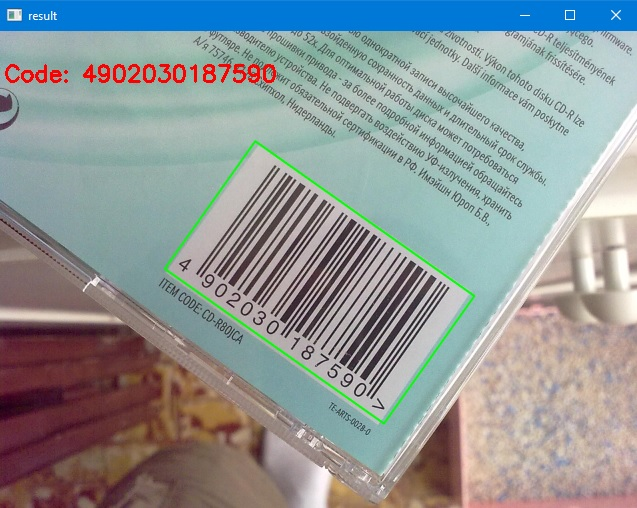## Benchmark and Limitations

A benchmark is run on dataset 1 in the Artelab Medium Barcode 1D Collection using this performance test tool.

The dataset contains 215 images taken by mobile phones.

We can see that it has a 57.21% accuracy and a 87.86% precision. It is fairly good but worse than commercial and open-source barcode reading libraries. Its processing speed is also slow, which takes about 3.5 seconds to decode an image.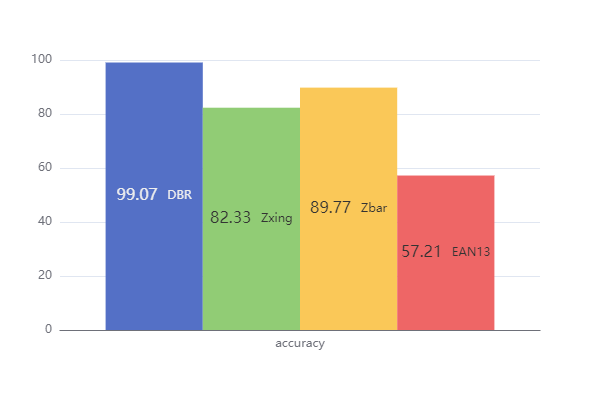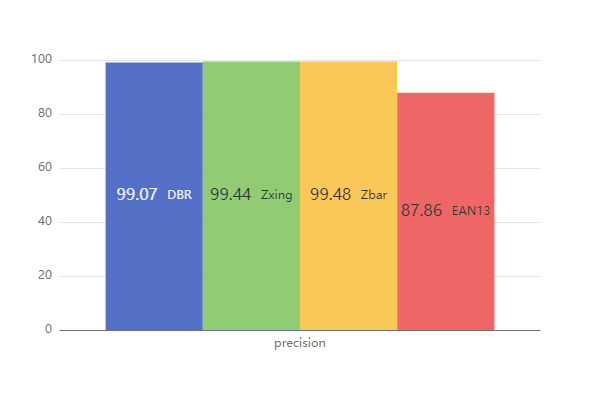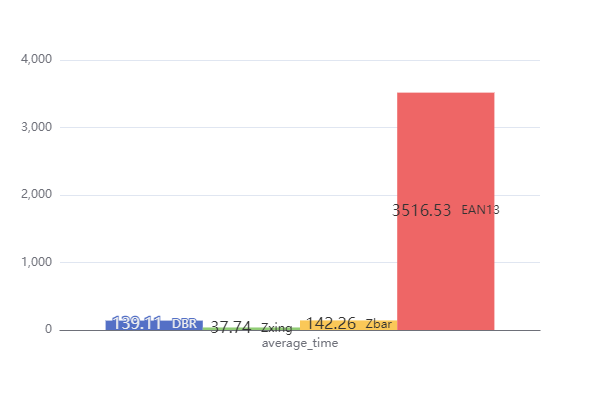By observing what it cannot read, we can find its limitations.

1. It cannot read flipped barcodes.
3. It cannot read barcodes without enough quiet zones.
4. It cannot read deformed barcodes.

Dynamsoft Barcode Reader (DBR) is a sophisticated barcode reading SDK which can read 1D and 2D barcodes even in various bad conditions. In the benchmark, only Dynamsoft Barcode Reader can read images like the following one.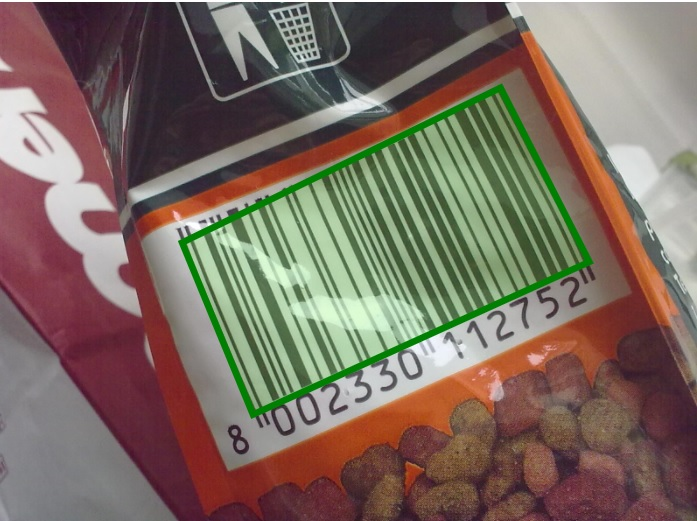### Why Choose Dynamsoft Barcode Reader

Here are the highlights of why you should choose Dynamsoft Barcode Reader: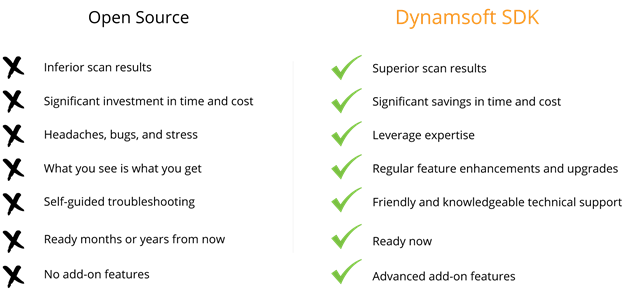• Powerful barcode decoding can scan over 50 barcodes at once
• Exceptional performance in various usage scenarios
• Decodes problematic barcodes from out-of-focus, skewed, wrinkled, curved, glare, distorted, grainy, poor contrast and more
• Detects barcodes at any orientation and rotation angle
• 100+ APIs to enable advanced customization
• Supports multiple platforms — iOS, Android, Windows, Linux, Web, Raspberry Pi

If you’re at the stage where you’re testing different options, try Dynamsoft Barcode Reader online demo or download a 30-day free trial. There’s no commitment necessary.

Dynamsoft was founded in 2003 in Vancouver, Canada. Since then, we have earned the trust of many Fortune 500 companies, including Lockheed Martin, HP, IBM, Intel, Disney, the US Government, NASA, Siemens, and many more.

Try Online Barcode Scanner Demo